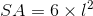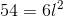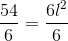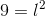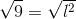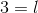# High School Math : How to find the length of an edge of a cube

## Example Questions

### Example Question #1 : How To Find The Length Of An Edge Of A Cube

Our backyard pool holds 10,000 gallons.  Its average depth is 4 feet deep and it is 10 feet long.  If there are 7.48 gallons in a cubic foot, how wide is the pool?

100 ft

30 ft

133 ft

33  ft

7.48 ft

33  ft

Explanation:

There are 7.48 gallons in cubic foot. Set up a ratio:

1 ft3 / 7.48 gallons = x cubic feet / 10,000 gallons

Pool Volume = 10,000 gallons = 10,000 gallons * (1 ft3/ 7.48 gallons) = 1336.9 ft3

Pool Volume = 4ft x 10 ft x WIDTH = 1336.9 cubic feet

Solve for WIDTH:

4 ft x 10 ft x WIDTH = 1336.9 cubic feet

WIDTH = 1336.9 / (4 x 10) = 33.4 ft

### Example Question #2 : How To Find The Length Of An Edge Of A Cube

A cube has a volume of 64cm3. What is the area of one side of the cube?

16cm2

16cm3

4cm2

16cm

4cm

16cm2

Explanation:

The cube has a volume of 64cm3, making the length of one edge 4cm (4 * 4 * 4 = 64).

So the area of one side is 4 * 4 = 16cm2

### Example Question #3 : How To Find The Length Of An Edge Of A Cube

Given that the suface area of a cube is 72, find the length of one of its sides.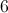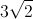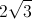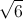Explanation:

The standard equation for surface area is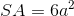wheredenotes side length. Rearrange the equation in terms ofto find the length of a side with the given surface area: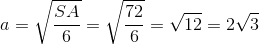### Example Question #4 : How To Find The Length Of An Edge Of A Cube

Find the length of an edge of the following cube: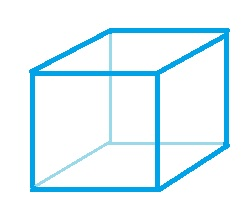The volume of the cube is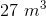.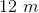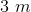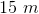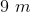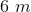Explanation:

The formula for the volume of a cube is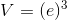,

where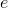is the length of the edge of a cube.

Plugging in our values, we get: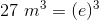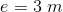### Example Question #5 : How To Find The Length Of An Edge Of A Cube

Find the length of an edge of the following cube:The volume of the cube is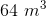.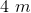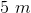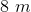Explanation:

The formula for the volume of a cube is,

whereis the length of the edge of a cube.

Plugging in our values, we get: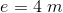### Example Question #6 : How To Find The Length Of An Edge Of A Cube

What is the length of an edge of a cube that has a surface area of 54?Explanation:

The surface area of a cube can be determined using the following equation: